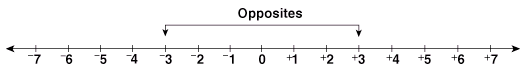# Absolute Value

Problem: Jill and Juan were at Amy's house. Jill rode her bicycle 3 miles west of Amy's house, and Juan rode his bicycle 3 miles east of Amy's house. Who traveled a greater distance from Amy's house - Jill or Juan?

Solution: Jill and Juan both traveled the same distance from Amy's house since each traveled 3 miles (in opposite directions).

The problem above can be solved using integers. Traveling 3 miles west can be represented by -3 (pronounced negative 3). Traveling 3 miles east can be represented by +3 (pronouncedpositive 3). Amy's house can be represented by the integer 0.Two integers that are the same distance from zero in opposite directions are called opposites. The integers +3 and -3 are opposites since they are each 3 units from zero.The absolute value of an integer is its distance from zero on the number line. The absolute value of +3 is 3, and the absolute value of -3 is 3. Thus, opposite integers have the same absolute value. Mathematicians use the symbol || for absolute value. Let's look at some examples.

Example 1: Find the absolute value of  +3,  -3,   +7,   -5,  +9,  -8,  +4,  -4.   You may refer to the number line below.Solution:

 |+11| = 11 |+17| = 17 |-9| = 9 |-19| = 19 |+14| = 14 |+20| = 20 |-10| = 10 |-20| = 20

Remember that when we find the absolute value of an integer, we are finding its distance from 0 on the number line. Opposite integers have the same absolute value since they are both the same distance from 0. Also, you will notice that taking the absolute value of an integer strips the sign from that integer.

Example 2: Find the absolute value of:  +11,  -9,   +14,   -10,  +17,  -19,  +20,  -20.   You may extend the number line below to help you solve this problem.Solution:

 |+11| = 11 |+17| = 17 |-9| = 9 |-19| = 19 |+14| = 14 |+20| = 20 |-10| = 10 |-20| = 20

Example 3: Use the number line below to solve this problem: |x| = 5Solution: This problem asks us to find all integers that are a distance of 5 units from zero on the number line. We let x represent all integers that satisfy this condition.

The integer +5 is 5 units from zero on the number line, and the integer -5 is also 5 units from zero on the number line. Thus both +5 and -5 satisfy the given condition.

x = +5  and  x = -5, or we can write x = {+5, -5}

Summary: The absolute value of an integer is its distance from zero on the number line. The notation |x| is used for the absolute value of an unknown integer that satisfies a given condition.

### Exercises

Directions: Read each question below. Click once in an ANSWER BOX and type in your answer; then click ENTER. After you click ENTER, a message will appear in the RESULTS BOX to indicate whether your answer is correct or incorrect. To start over, click CLEAR.

 1 Find the absolute value of -6. ANSWER BOX:   RESULTS BOX:
 2 Find |+15| ANSWER BOX:   RESULTS BOX:
 3 Find |-31| ANSWER BOX:   RESULTS BOX:
 4 Find |+31| ANSWER BOX:   RESULTS BOX:
 5 How many values of y will you get when you solve |y| = 29? ANSWER BOX:   RESULTS BOX: